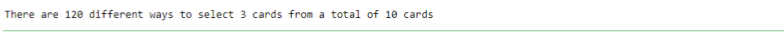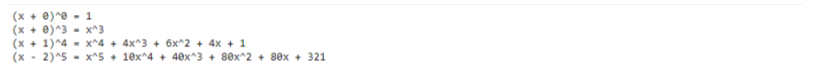## math.comb Python

##### Aniekan

To simplify the calculation of combinations, Python provides a library function, math.comb, which prevents us from writing complex code. Combinations involve selecting items from a collection without considering the order in which they are chosen.

In this article, we will discuss more about the Python math.com function, and how we could make use of it in another function that carries out binomial expansion.

## Understanding math.comb function in Python

To calculate combinations without writing the code ourselves,  Python provides us with themath.comb function.

math.comb  function calculates and returns an integer representing the number of ways we can select items from a collection without bothering about the order in which the items are selected.

Generally, we call math.comb function as follows:

math.comb(n, k)or:


from math import comb

comb(n, k)


The parameter nis a positive integer representing the size of the collection, and k is a positive integer representing the number of items to choose from the collection.

When math.comb is called, it returns the number of possible ways to select k items from a set having n items.

However, we have to note the following behaviors of math.comb:

1. math.comb will return 0 if k is greater than n
2. A ValueError will be raised if either n or k (or both) are negative.
3. A TypeError will be raised if either parameter is not an int

The following code snippets show how to make use of Python math.comb function.

### Example: Selecting playing cards

If we had a set of 10 playing cards, and we wanted to find out the number of different ways we can select 3 cards from the set.

We can make use of the following Python code to carry out the calculation.

from math import comb
total_num_of_cards = 10
num_to_select = 3

result = comb(total_num_of_cards, num_to_select)

print(f"There are {result} different ways to select {num_to_select} cards from a total of {total_num_of_cards} cards")


The code snippet effectively makes the function call math.comb(10, 3) to get the number of different ways to make the required selection.

The output is shown below:Now that we have seen how to make use of the math.comb function in Python.

We will now look into one application; using math.comb function to calculate binomial coefficients.

First, we will discuss the binomial expansion before diving into its Python implementation.

## The Binomial Expansion of (x+a)^n

The binomial expansion allows us to expand a binomial expression raised to a non-negative integer power.

That is, using binomial expansion, we can remove brackets from expressions having the form (x+a)^n, where n is a non-negative integer, and a is a constant, and rewrite it into a polynomial expression.

After expanding (x+a)^n, the coefficients of each term in the resulting polynomial are binomial coefficients.

In other words, each term in the polynomial will have the form C(n, k)x^{n-k}a^k.

The factor C(n, k) is the binomial coefficient, and is calculated using the combination formula \frac{n!}{(n-k)!k!}. As a result of this connection with Python’s math.comb function, we can easily create functionality to carry out binomial expansion for us.

This will be examined in the next section.

## Using Python math.comb for binomial expansion of (x+a)^n

We want to implement a simple function that will take as input the values a and n, and then compute and return the binomial expansion for the specified parameters.

The code is shown below.

from math import comb

# This function converts the input coefficient to a string
def get_coeff_str(coeff):
return str(coeff) if coeff>1 else ""

# This function determines the sign of a term
def get_sign_str(parity):
return '+' if parity==1 else '-'

# This function returns the string representation of x raised to power m
def get_pow_str(m):
return "1" if m==0 else ("x" if m==1 else f"x^{m}")

# Main function to expand (x + a)^n
def expand(a, n):
terms = []

# If a is zero, just return a simple expression for the expansion
if a==0:
return get_pow_str(n)

# Construct all the terms
for i in range(n+1):
# Compute the coefficient for the current term
coeff = int(abs(comb(n, i)*a**i))

# Get the sign for the current term
parity = int(coeff/abs(coeff))

# Get the power/index of factor x for the current term
idx_x = n-i

# Construct the current term as a tuple
term = parity, coeff, idx_x

# Append the current term to the list of terms
terms.append(term)

# Get the string representation of the first term
idx_1 = terms
first_term = get_pow_str(idx_1)

# Get the string representation of the remaining terms
other_terms = "".join(
[
f" {get_sign_str(parity)} {get_coeff_str(coeff)}{get_pow_str(idx_x)}"
for parity, coeff, idx_x in terms[1:]
]
)

# Return the final representation of the expanded binomial
return first_term + other_terms


Our code contains functions that, together, help produce the string representation of a binomial expansion when given values of a and n. The main function is expand which accepts parameters a and n, and returns the string representing the expansion (x+a)^n.

The function expandchecks for the special case a==0, which, if it occurs, will just call get_power_str to return the  m_{th} power of x.

If a is not zero, it first constructs a list of term objects, each term being represented in memory as a tuple (parity, coeff, idx_x).

Then it constructs the string expression of the final polynomial from the list of term objects.

We can see that while constructing the binomial coefficient for the current term in the for loop, it makes use of math.comb.

Our code listing has several helper functions to help create the string representation for each term:

• get_coeff_str(coeff): Returns the string representation for the coefficient coeff. It takes care of the special case coeff==1, in which case it returns an empty string. With this function in place, we can avoid representations like 1xor 1x^3.
• get_sign_str(parity): Returns the sign for the term. The parity can be 1 or -1, which will correspond to + or - signs respectively.
• get_power_str(m): Returns the string representation for the m_{th} power of x. It takes care of cases like m==0 (which would lead to representations like x^0), and m==1(which leads to representations like x^1).

To test the code, we can use the following lines:

# Example usage:
print(f"(x + 0)^0 = {expand(0, 0)}")
print(f"(x + 0)^3 = {expand(0, 3)}")
print(f"(x + 1)^4 = {expand(1, 4)}")
print(f"(x - 2)^5 = {expand(-2, 5)}")

The following output is displayed:The output contains cases where a = 0 ((x+0)^0 and (x+0)^3), and their corresponding expansions.

We can also see sample expansions for (x + 1)^4 and (x + 1)^4.

Python math.comb proved to be a valuable tool in our small program that creates the string representation for the binomial expansion of (x + a)^n.

This simplified our code, instead of us having to create our own definition for calculating binomial coefficients. With this function provided by Python math module, we can write code that implements powerful combinatorial and algebraic capabilities.

We have come to the end of our tutorial. We believe you learned something useful that can help you in your coding journey. Feel free to check out other interesting Python tutorials.

.